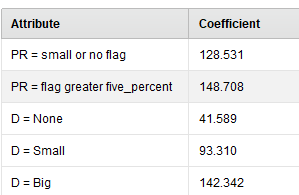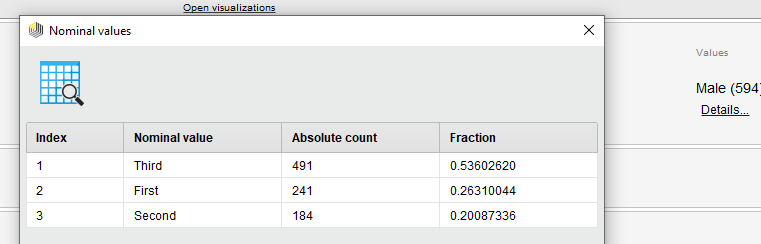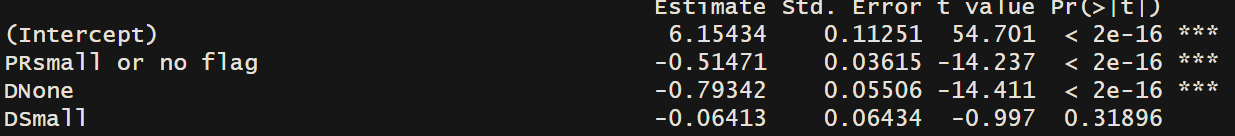# Reference Category in Linear Regression

Member Posts: 45Guru
Hello everyone,
although I searched the forum, I did not find anything applicable for my cas. If have overlooked something, I am sorry.
The Linear Regression model gives me the following result:I would like to have D = NONE as a reference-category so that it would not be inside the result.

(How) is that possible?

Have a nice day and weekendTagged:

• Administrator, Employee, RapidMiner Certified Analyst, RapidMiner Certified Expert, Member Posts: 363RM Data Scientist
edited January 2020
Hi @MPB_,

to change the reference/baseline category in linear regression, you can manually reorder the example set. The baseline category is determined by the appearance order. The first appeared nominal value in data is chosen to be the reference category. For instance in Titanic data, after some re-ordering, my statistics summary for categorical factors (details for counts of nominal values) has an updated over view:The process xml that change the example order and update the model with new reference category ---
```<?xml version="1.0" encoding="UTF-8"?><process version="9.5.001">
<context>
<input/>
<output/>
<macros/>
</context>
<operator activated="true" class="process" compatibility="9.5.001" expanded="true" name="Process">
<parameter key="logverbosity" value="init"/>
<parameter key="random_seed" value="2001"/>
<parameter key="send_mail" value="never"/>
<parameter key="process_duration_for_mail" value="1"/>
<parameter key="encoding" value="SYSTEM"/>
<process expanded="true">
<operator activated="true" class="retrieve" compatibility="9.5.001" expanded="true" height="68" name="Retrieve Titanic Training" width="90" x="45" y="34">
<parameter key="repository_entry" value="//Samples/data/Titanic Training"/>
</operator>
<operator activated="true" class="multiply" compatibility="9.5.001" expanded="true" height="103" name="Multiply" width="90" x="179" y="34"/>
<operator activated="true" class="filter_example_range" compatibility="9.5.001" expanded="true" height="82" name="Filter Example Range" width="90" x="313" y="34">
<parameter key="first_example" value="473"/>
<parameter key="last_example" value="474"/>
<parameter key="invert_filter" value="false"/>
</operator>
<operator activated="true" class="filter_example_range" compatibility="9.5.001" expanded="true" height="82" name="Filter Example Range (2)" width="90" x="447" y="136">
<parameter key="first_example" value="473"/>
<parameter key="last_example" value="474"/>
<parameter key="invert_filter" value="true"/>
</operator>
<operator activated="true" class="append" compatibility="9.5.001" expanded="true" height="103" name="Append" width="90" x="581" y="34">
<parameter key="datamanagement" value="double_array"/>
<parameter key="data_management" value="auto"/>
<parameter key="merge_type" value="all"/>
</operator>
<operator activated="true" class="h2o:generalized_linear_model" compatibility="9.3.001" expanded="true" height="124" name="Generalized Linear Model" width="90" x="715" y="34">
<parameter key="family" value="AUTO"/>
<parameter key="solver" value="AUTO"/>
<parameter key="reproducible" value="false"/>
<parameter key="use_regularization" value="false"/>
<parameter key="lambda_search" value="false"/>
<parameter key="number_of_lambdas" value="0"/>
<parameter key="lambda_min_ratio" value="0.0"/>
<parameter key="early_stopping" value="true"/>
<parameter key="stopping_rounds" value="3"/>
<parameter key="stopping_tolerance" value="0.001"/>
<parameter key="standardize" value="true"/>
<parameter key="non-negative_coefficients" value="false"/>
<parameter key="compute_p-values" value="false"/>
<parameter key="remove_collinear_columns" value="false"/>
<parameter key="missing_values_handling" value="MeanImputation"/>
<parameter key="max_iterations" value="0"/>
<parameter key="specify_beta_constraints" value="false"/>
<list key="beta_constraints"/>
<parameter key="max_runtime_seconds" value="0"/>
<list key="expert_parameters"/>
</operator>
<operator activated="true" class="h2o:generalized_linear_model" compatibility="9.3.001" expanded="true" height="124" name="Generalized Linear Model (2)" width="90" x="313" y="340">
<parameter key="family" value="AUTO"/>
<parameter key="solver" value="AUTO"/>
<parameter key="reproducible" value="false"/>
<parameter key="use_regularization" value="false"/>
<parameter key="lambda_search" value="false"/>
<parameter key="number_of_lambdas" value="0"/>
<parameter key="lambda_min_ratio" value="0.0"/>
<parameter key="early_stopping" value="true"/>
<parameter key="stopping_rounds" value="3"/>
<parameter key="stopping_tolerance" value="0.001"/>
<parameter key="standardize" value="true"/>
<parameter key="non-negative_coefficients" value="false"/>
<parameter key="compute_p-values" value="false"/>
<parameter key="remove_collinear_columns" value="false"/>
<parameter key="missing_values_handling" value="MeanImputation"/>
<parameter key="max_iterations" value="0"/>
<parameter key="specify_beta_constraints" value="false"/>
<list key="beta_constraints"/>
<parameter key="max_runtime_seconds" value="0"/>
<list key="expert_parameters"/>
</operator>
<connect from_op="Retrieve Titanic Training" from_port="output" to_op="Multiply" to_port="input"/>
<connect from_op="Multiply" from_port="output 1" to_op="Filter Example Range" to_port="example set input"/>
<connect from_op="Multiply" from_port="output 2" to_op="Generalized Linear Model (2)" to_port="training set"/>
<connect from_op="Filter Example Range" from_port="example set output" to_op="Append" to_port="example set 1"/>
<connect from_op="Filter Example Range" from_port="original" to_op="Filter Example Range (2)" to_port="example set input"/>
<connect from_op="Filter Example Range (2)" from_port="example set output" to_op="Append" to_port="example set 2"/>
<connect from_op="Append" from_port="merged set" to_op="Generalized Linear Model" to_port="training set"/>
<connect from_op="Generalized Linear Model" from_port="model" to_port="result 1"/>
<connect from_op="Generalized Linear Model" from_port="exampleSet" to_port="result 2"/>
<connect from_op="Generalized Linear Model (2)" from_port="model" to_port="result 3"/>
<portSpacing port="source_input 1" spacing="0"/>
<portSpacing port="sink_result 1" spacing="0"/>
<portSpacing port="sink_result 2" spacing="0"/>
<portSpacing port="sink_result 3" spacing="252"/>
<portSpacing port="sink_result 4" spacing="0"/>
</process>
</operator>
</process>```
• Member Posts: 45Guru
Hi @yyhuang,
thank you very much for your reply - this is very nice to know.

Nevertheless, I think I was not specific enough. What I would expect is, that there would be no estimates / values for the reference category. For example in my case, I would expect the level D = "NONE" to be not in the results or with a value of 0 or 1.

In your case, I would expect the Level "First" to be not in the results or with a value of 0 or 1.

I hope you have a nice weekend.

• Member Posts: 45Guru
Edit: The reason why I am asking this is that other softwares such as RStudio and IBM SPSS behave in that way.

If I run the same structured data through RStudio, you can see that for example "PR flag greater five percent" and D = "Big" were taken as the reference-levels and are not inside the results / have no estimates.